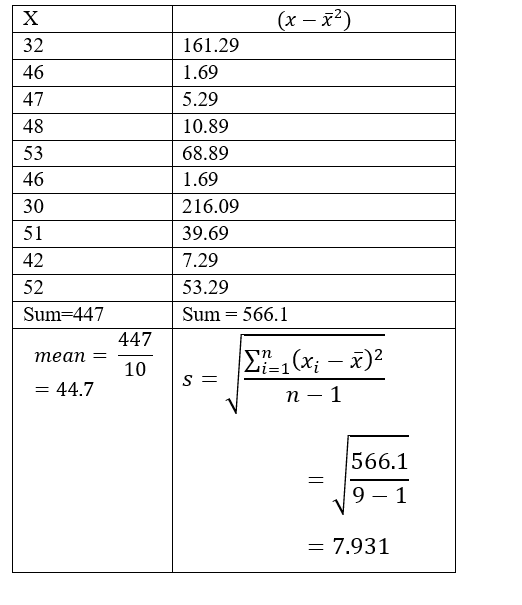Question
1 views

The manufacturer of a sports car claims that the fuel injection system lasts 48 months before it needs to be replaced. A consumer group tests this claim by surveying a random sample of 10 owners who had the fuel injection system replaced. The ages of the cars at the time of replacement were (in months):
32 46 47 48 53 46 30 51 42 52
(i) Use your calculator to calculate the mean age of a car when the fuel injection system fails x and standard deviation s. (Round your answers to two decimal places.)
x =
44.7

months
s = 10
months

(ii) Test the claim that the fuel injection system lasts less than an average of 48 months before needing replacement. Use a 5% level of significance.
What are we testing in this problem?
single mean
single proportion

(a) What is the level of significance?
0.05

State the null and alternate hypotheses.
H0: μ = 48; H1: μ ≠ 48
H0: μ = 48; H1: μ > 48
H0: p = 48; H1: p < 48
H0: p = 48; H1: p ≠ 48
H0: μ = 48; H1: μ < 48
H0: p = 48; H1: p > 48

(b) What sampling distribution will you use? What assumptions are you making?
The standard normal, since we assume that x has a normal distribution with known σ.
The Student's t, since we assume that x has a normal distribution with unknown σ.
The standard normal, since we assume that x has a normal distribution with unknown σ.
The Student's t, since we assume that x has a normal distribution with known σ.

What is the value of the sample test statistic? (Round your answer to three decimal places.)
-2.334

.2345

Sketch the sampling distribution and show the area corresponding to the P-value.
WebAssign Plot WebAssign Plot
WebAssign Plot WebAssign Plot

(d) Based on your answers in parts (a) to (c), will you reject or fail to reject the null hypothesis? Are the data statistically significant at level α?
At the α = 0.05 level, we reject the null hypothesis and conclude the data are statistically significant.
At the α = 0.05 level, we reject the null hypothesis and conclude the data are not statistically significant.
At the α = 0.05 level, we fail to reject the null hypothesis and conclude the data are statistically significant.
At the α = 0.05 level, we fail to reject the null hypothesis and conclude the data are not statistically significant.

(e) Interpret your conclusion in the context of the application.
There is sufficient evidence at the 0.05 level to conclude that the injection system lasts less than an average of 48 months.
There is insufficient evidence at the 0.05 level to conclude that the injection system lasts less than an average of 48 months.

check_circle

Step 1

Hello there! there are more than three subparts in the question. According to our policies we cannot answer more than three sub parts in one question. So please find the solution for a,b,c parts of the question. If you have doubts in remaining part of the question kindly make a new request, mentioning the part in which you need help

i)

Given data and calculation for mean and standard deviation is shown belowStep 2

Mean = 44.7

Standard deviation = 7.931

ii)

We are testing single mean

Level of significance = 0.05

Formation of Hypothesis

Null Hypothesis H0: μ = 48;

Alternative Hypothesis  H1: μ < 48

b)

Since population deviation is unknown, we will use student’s t-distribution

The Student's t, since we assume that x has a normal distribution with unknown &...

### Want to see the full answer?

See Solution

#### Want to see this answer and more?

Solutions are written by subject experts who are available 24/7. Questions are typically answered within 1 hour.*

See Solution
*Response times may vary by subject and question.
Tagged in